# Solution of Plasma Angular Equations of Motion

In many situations of interest, the perturbed angular velocity profiles,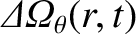and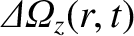, are localized in the vicinity of the rational surface . Hence, it is reasonable to express the perturbed angular equations of motion, (3.161) and (3.165), in the simplified forms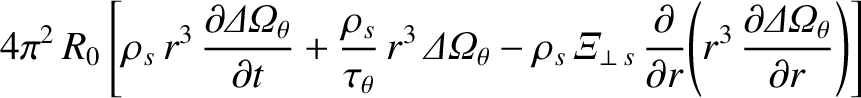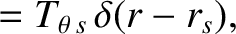(3.168)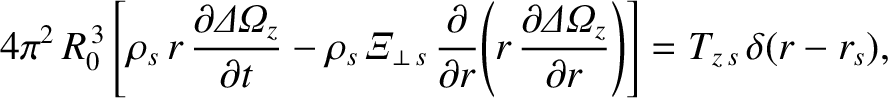(3.169)

where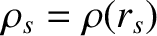,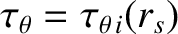, and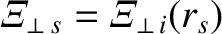.

Let us write [3,5]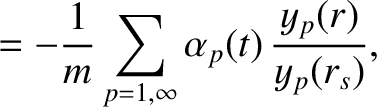(3.170)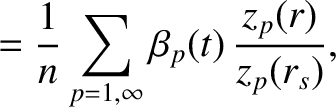(3.171)

where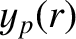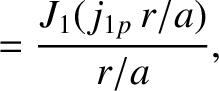(3.172)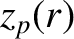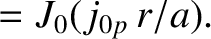(3.173)

Here,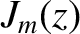is a Bessel function, and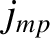denotes its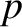th zero . Note that Equations (3.170)–(3.173) automatically satisfy the boundary conditions (3.166) and (3.167).

It is easily demonstrated that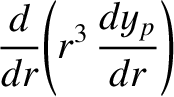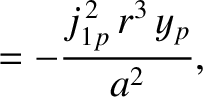(3.174)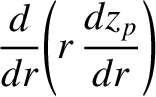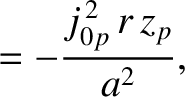(3.175)

and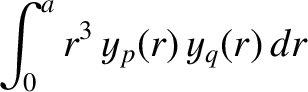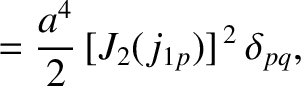(3.176)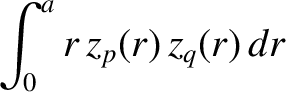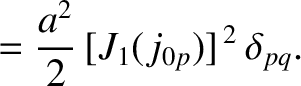(3.177)

Equations (3.139), (3.140), and (3.168)–(3.177) yield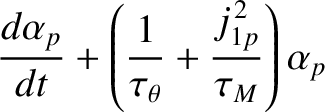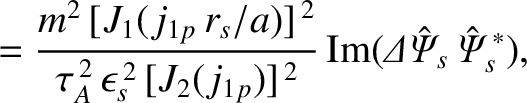(3.178)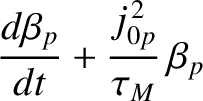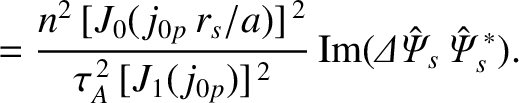(3.179)

Here,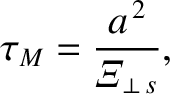(3.180)

is the momentum confinement time,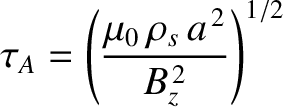(3.181)

is the Alfvén time,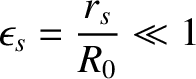(3.182)

is the inverse aspect-ratio of the rational surface, and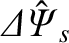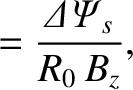(3.183)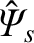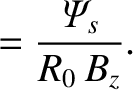(3.184)